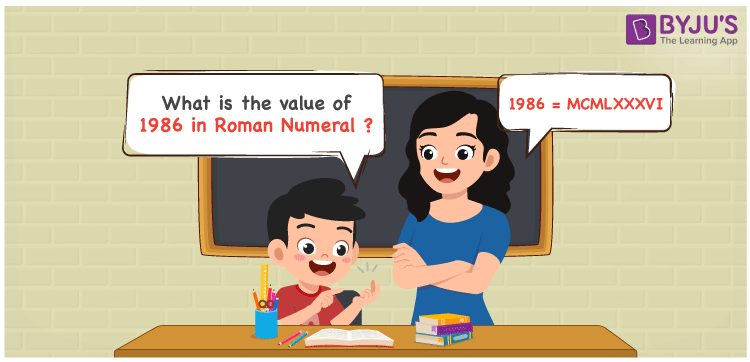# 1986 in Roman Numerals

1986 in Roman Numerals is MCMLXXXVI. Any Roman numeral uses only the 7 primary symbols which are I, V, X, L, C, D, and M, Where M represents 1000, C represents 100, L represents 50, X represents 10 and I represents 1. MCMLXXXVI represents the number 1986 which is obtained by the addition and subtraction rules of Roman Numerals. What are Roman numerals? can be referred for more details.

 Number Roman Numeral 1986 MCMLXXXVI

## How to Write 1986 in Roman Numerals?To convert 1986 in Roman Numerals, we need to represent 1986 as the sum of the fundamental symbols. 1986 can be broken down as

1. The number 1000,
2. Difference of 1000 and 100 to get 900
3. Add 50, to thrice of 10 to get 80
4. Add 1 with 5 to get 6
5. Add all the numbers from previous steps.

Let us understand it with Numerical representation.

1986 = 1000 + 900 + 80 + 6

1986 = 1000 + (1000 – 100) + (50 + 10 + 10 + 10) + ( 5 + 1)

1986 = M + (M – C) + (L + X + X + X) + (V + 1)

1986 = M + (CM) + LXXX + VI

1986 = MCMLXXXVI

## Video Lesson on Roman Numerals## Frequently Asked Questions on 1986 in Roman Numerals

Q1

### How to write the number 1986 in Roman Numerals?

1986 in Roman Numerals is written as MCMLXXXVI.
Q2

### Can V be repeated thrice?

No. V cannot be repeated.
Q3

### What is the year 1986 in Roman numerals?

The year 1986 in Roman Numerals is MCMLXXXVI.